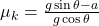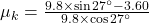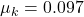## A student solving a physics problem to find the unknown has applied physics principles and obtained the expression: μkmgcosθ=mgsinθ−ma, wher

Question

A student solving a physics problem to find the unknown has applied physics principles and obtained the expression: μkmgcosθ=mgsinθ−ma, where g=9.80meter/second2, a=3.60meter/second2, θ=27.0∘, and m is not given. Which of the following represents a simplified expression for μk?A student solving a physics problem to find the unknown has applied physics principles and obtained the expression: , where , , , and is not given. Which of the following represents a simplified expression for ?tanθ− agTo avoid making mistakes, the expression should not be simplified until the numerical values are substituted.gsinθ−agcosθThe single equation has two unknowns and cannot be solved with the information given.

in progress 0
6 months 2021-07-19T04:32:28+00:00 1 Answers 106 views 0

1. Solution :

Given expression :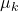mgcosθ = mgsinθ − ma

Here, g = 9.8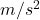, a = 3.60, θ = 27°

Therefore,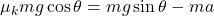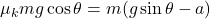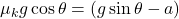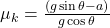Mow calculating the coefficient of kinetic friction as follows :